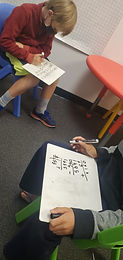## Mr. Jake

### Target 1​

###### Lesson Type:

Continuation

Measurment

:

Comparison

Measure and compare objects in standard units.

###### 1:

Understand how to measure lengths with fractional increments.

5th

###### Vocabulary:

Fraction, Unit

Activities:

Used fractional measurments to measure objects around the room accuratly

Identified what fraction smaller units represent in a larger unit (1 inch is 1/12 of a foot)### Home Exploration

###### Guiding Questions:## Absent Students:

### Target 2

:

###### 1:

Define each type of quadrilateral (square, rectangle, parallelogram, rhombus, and trapezoid).

###### 2:

Identify parallel and perpendicular lines.

###### 3:

Find the hierarchical relationship between the different types of quadrilaterals.

7th

###### Vocabulary:

Activities:

Discussed how to classify different quadrilaterals

Created the hierarchy of quadrilaterals using a tree diagram.### Home Exploration

###### Guiding Questions:### Target 3

:

###### 1:

Solve problems that have more than one operation.

###### 2:

Understand that the presence of parenthesis in an arithmetic equation signals for this part of the problem to be solved first.

###### 3:

Understand that the order of operations must be followed to evaluate expressions.

###### 4:

Understand that calculating in the wrong order can result in a wrong answer.

###### 5:

Define PEMDAS – Parenthesis, Exponents, Multiplication, Division, Addition, Subtraction.

7th

###### Vocabulary:

Activities:

Practiced solving multistep equations using PEMDAS### Home Exploration